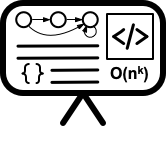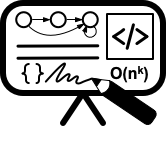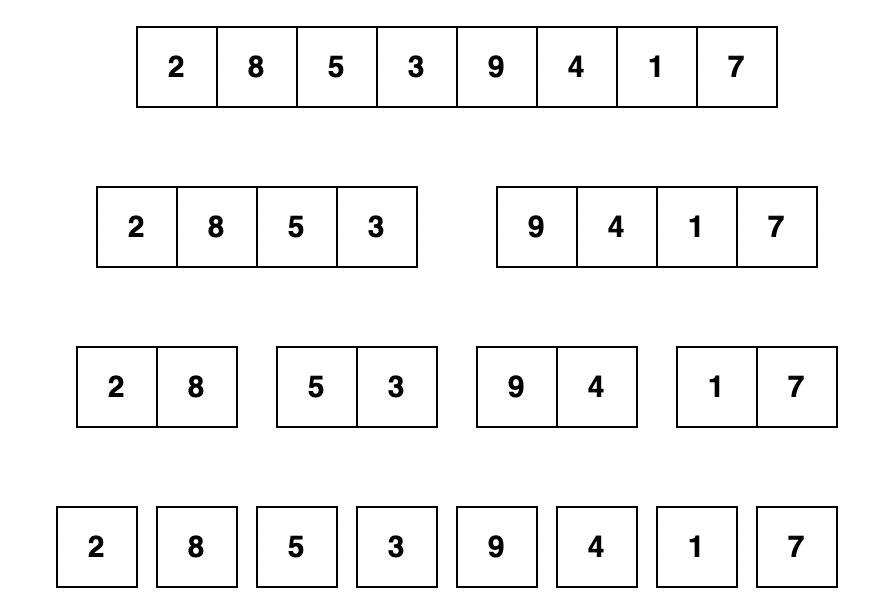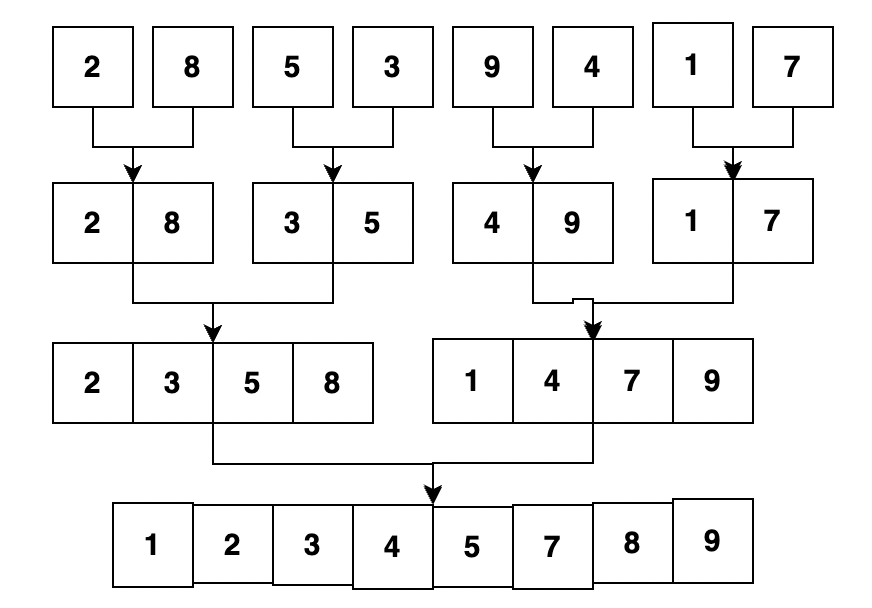# Lecture 10 - Divide and conquer and selection algorithms

Date Pre-lecture slides Post-lecture scribbles Lecture recording
September 28 2023## Introduction to Divide and Conquer Algorithms

Divide and Conquer is a popular algorithmic paradigm used in computer science and mathematics. It is a problem-solving technique that breaks a complex problem into smaller sub-problems, solves each sub-problem independently, and then combines their solutions to obtain the solution of the original problem.

The “divide” step involves breaking the problem into smaller sub-problems, while the “conquer” step involves solving each sub-problem. Finally, the “combine” step involves merging the solutions of the sub-problems to obtain the solution of the original problem.

This approach is often used to solve problems that can be broken down into smaller, simpler sub-problems, and is frequently used in computer science for algorithm design. Divide and conquer algorithms have been used to solve a wide range of problems, including sorting, searching, matrix multiplication, and many more. The efficiency and effectiveness of divide and conquer algorithms have made them an important tool in the arsenal of computer scientists and mathematicians.

## Merge Sort Algorithm

Merge Sort is a sorting algorithm that follows the Divide and Conquer paradigm. It works by recursively dividing the array into two halves until each sub-array contains only one element, and then merging the sorted sub-arrays to produce the final sorted array.

## Example:

Breaking down the array into simple single element arrays:After combining the small arrays by arranging elements in proper order:### Time Complexity

The time complexity of Merge Sort is O(n logn) in the average and worst cases, where n is the number of elements in the array. The space complexity of Merge Sort is O(n).

## Quick Sort Algorithm

Quick Sort is a sorting algorithm that follows the Divide and Conquer paradigm. It works by partitioning the array into three parts, based on a chosen pivot element, and recursively sorting the sub-arrays and concatenating them.

### Time Complexity

The time complexity of Quick Sort depends on the choice of pivot element. In the average case, the time complexity is O(nlogn), where n is the number of elements in the array. In the worst case, where the pivot element is always chosen to be the largest or smallest element, the time complexity is O(n^2). However, Quick Sort is usually faster than other sorting algorithms in practice, because it has good cache performance and can be easily parallelized.

## Quick Select Algorithm

Quickselect is a simple and efficient algorithm for finding the k-th smallest element in an unsorted array. The basic idea behind the algorithm is to use the partitioning technique from quicksort to divide the array into smaller and larger subarrays around a pivot element, and then recursively search only the subarray containing the desired element.

The algorithm has an average time complexity of O(n), where n is the size of the input array. However, the worst-case time complexity can be O(n^2), if the pivot element chosen is always the largest or smallest element in the array. This can be avoided by choosing a random or median-of-three pivot element.

### Improving Worst-Case Time Complexity of Quickselect

The worst-case time complexity of the quickselect algorithm can be improved from O(n^2) to O(n) by selecting a good pivot element.

### Median-of-Medians Algorithm

One way to select a good pivot element is to use the “median-of-medians” algorithm, which guarantees that the chosen pivot element is close to the true median of the subarray. The median-of-medians algorithm works by recursively dividing the subarray into groups of five elements, computing the median of each group, and then recursively computing the median of the medians until a single pivot element is found.

• Textbooks
• Erickson, Jeff. Algorithms
• Skiena, Steven. The Algorithms Design Manual
• Chapter 5 - Divide and Conquer
• Chapter 17.3 - Median and Selection
• Sedgewick, Robert and Wayne, Kevin. Algorithms (Forth Edition)
• Chapter 2.2 - MergeSort
• Chapter 2.3 - QuickSort
• Chapter 2.5 - Median and order statistics
• Cormen, Thomas, et al. Algorithms (Forth Edition)
• Chapter 4 - Divide and Conquer
• Chapter 14.4 - Longest Common Subsequence
• Sariel’s Lecture 11